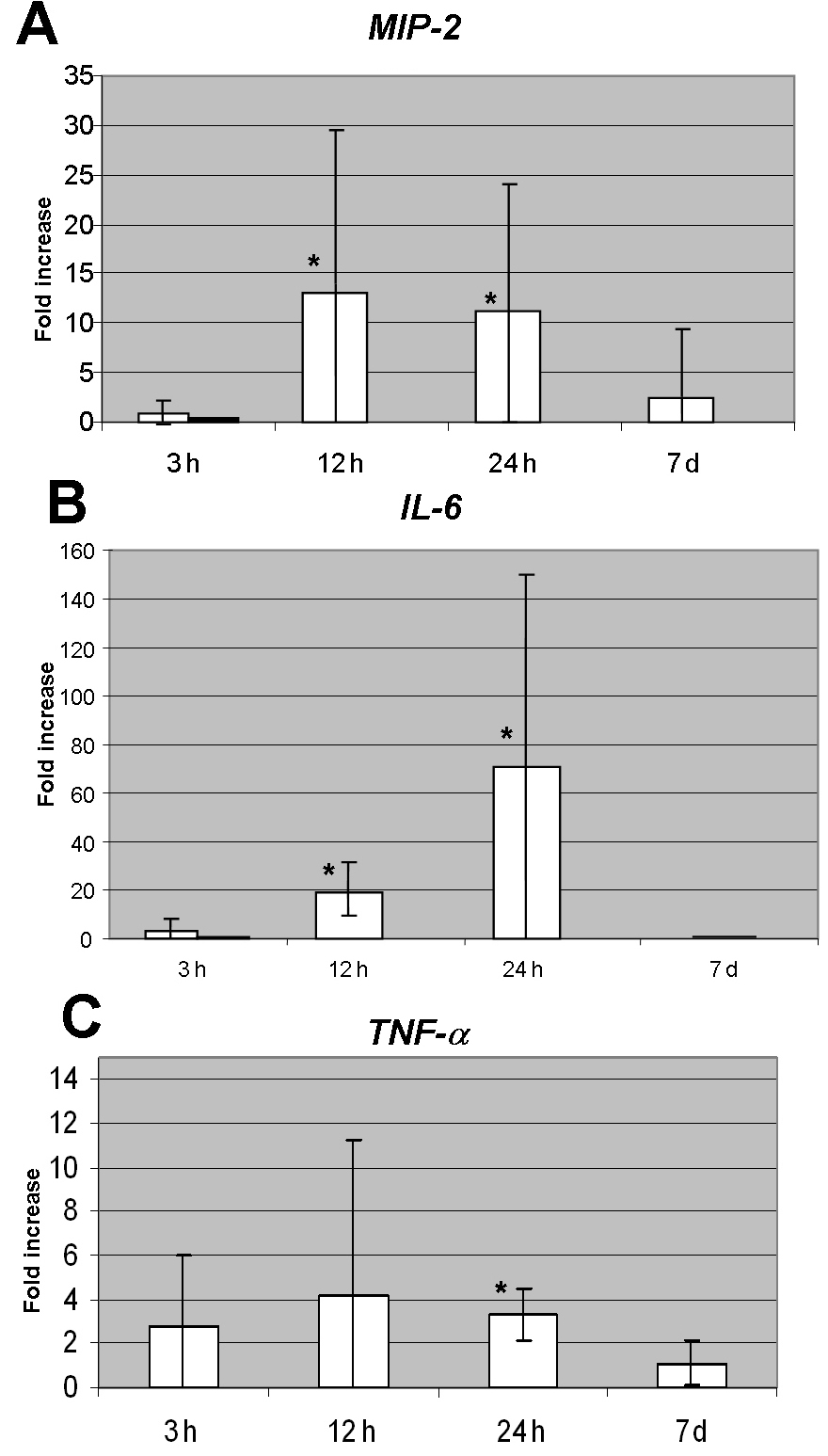Figure 1 of Kramer, Mol Vis 2009; 15:885-894.

Figure 1. Relative mRNA expression of proinflammatory cytokines in ischemic eyes compared to control eyes at variable time intervals from CRAO induction. A: Elevated levels of MIP-2 at 12 h and 24 h are statistically significantly higher than the levels at 3 h and 7 days (p<0.01). Light bar in the 3 h column represents total mean value, and the dark bar represents the trend of reduced expression. The bars at 12 h, 24 h, and 7 days display the mean values which represent the trends as well. B: Levels of IL-6 peaked at 12 h and 24 h. The differences between levels at 3 h and 12 h, as well as between 3 h and 24 h were statistically significant (p<0.01, 0.02, respectively). Levels at 7 days were statistically significantly lower than levels at 24 h (p<0.01). Light bar in the 3 h column represents total mean value, and the dark bar represents the trend of reduced expression. The bars at 12 h, 24 h, and 7 days display the mean values which represent the trends as well. C: Level of expression of TNF-α at 24 h was statistically significantly different from expression at 7 days (p=0.033). The asterisk indicates statistical significance. Error bars represent SD.